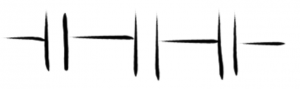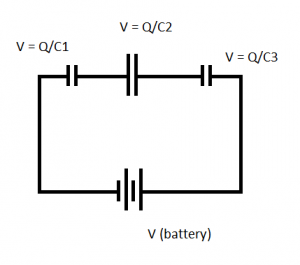# Capacitors in Series

• The charge is always the same for capacitors in series.
• Turn series capacitors into an equivalent capacitor like so:• These are eg. C1, C2, and C3
• Changing them into CEquivalent like so:1 / CEquiv = 1/C1 + 1/C2 + 1/C3 + ….

• The sum of the voltages across capacitors in series will equal the voltage of the battery

To calculate the voltage of capacitors:

Voltage across the capacitor (V) = Charge stored on a capacitor (Q) / Capacitance (C)

V = Q / C

• Q will remain constant in a series
• C depends on the capacitor• For the above image: Q/C1 + Q/C2 + Q/C3 = V (battery)
• Therefore: Q (1/C1 + 1/C2 + 1/C3) = V (battery)
• Finally we can deduce

1/C1 + 1/C2 + 1/C3 = V/Q = 1 / CEquivalent